# 木工榫头的计算公式，附：木工用到的140种榫卯结构详细图纸

###### 2020-02-18　来自: 甘肃富贵家具有限公司浏览次数:140

1、开口贯通单榫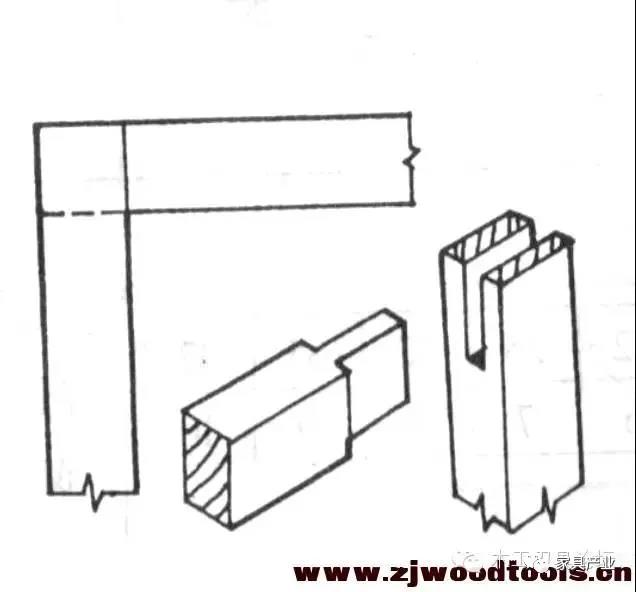s2=1/2x(s0-s1)      s2=1/2x(18-8)=5

2.开口贯通双水s0—开榫方材厚

s1—榫厚

s2—榫肩厚

s3—榫间距

当榫头位置对称时：

s1=s3=0.14xs0

s2=1/2x〔s0-(2xs1+s3) 〕

例：s0=45 求s1,s2,s3?

s1=s3=0.14x45=6.3  ～6

3，开口贯通三榫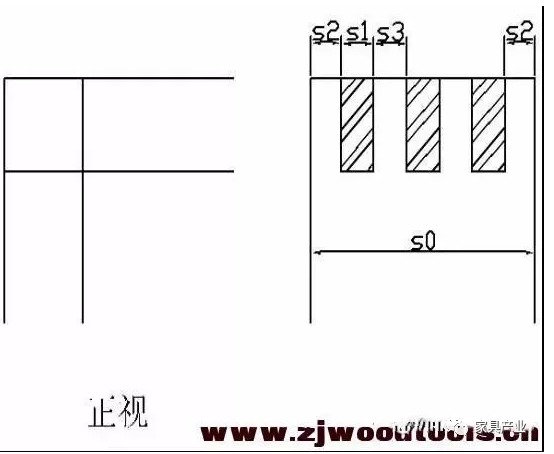s2=1/2x〔s0-(3xs1+2xs3) 〕

4.闭口不贯通三榫s1=(0.4~0.5)xs0

H=0.7xB1

L=(0.5~0.8)XB

s2=1/2X(S0-S1)

b :≥2mm

B\B1—方材宽度

S1=(0.4 ~0.5)x28=

s2=1/2x(28-s1)=

H=0.7x30=                L= (0.5~0.8)x30=

5.闭口贯通单榫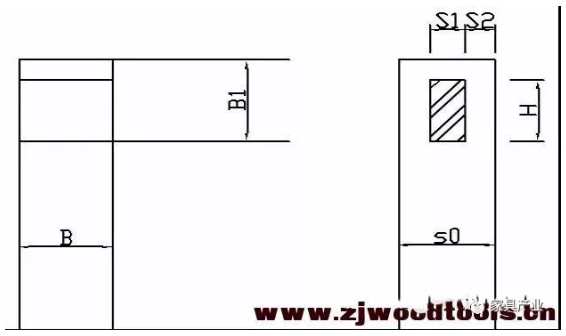s1=(0.4~0.5)xs0

H=0.6xB1

s2=1/2X(S0-S1)

S1=(0.4 ~0.5)x28=       s2=1/2x(28-s1)=

H=0.6x30=

[size=10.5000pt]

6.半开口不贯通单榫S1=(0.4~0.5)xs0

S2=1/2x(s0-s1)

H=0.7xB1

L=(0.5~0.8)XB

L1=(0.3~0.6)xB

b ≥ 2mm

S1=(0.4 ~0.5)x28=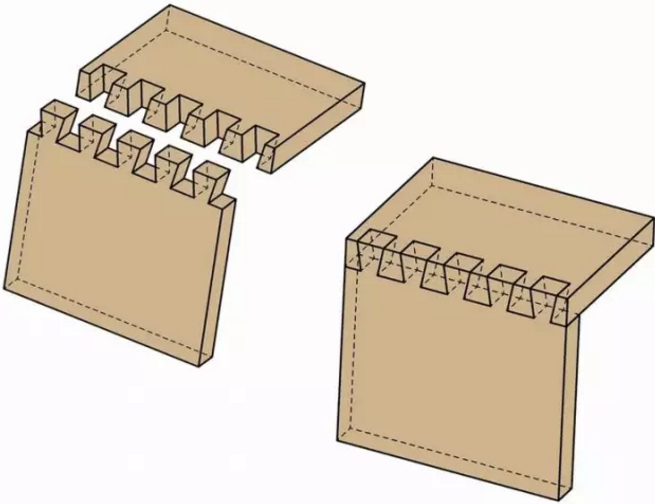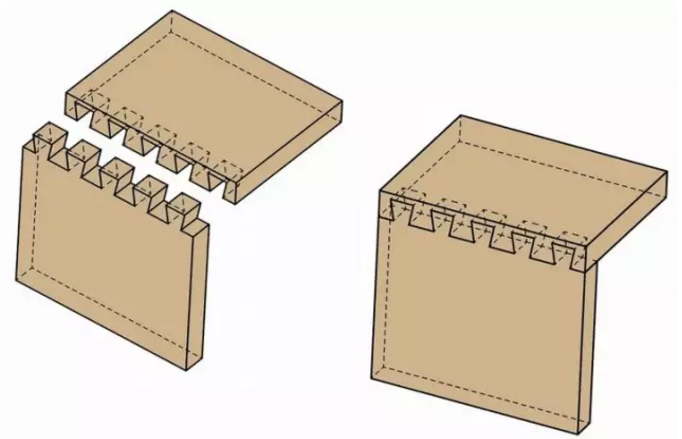全隐燕尾榫：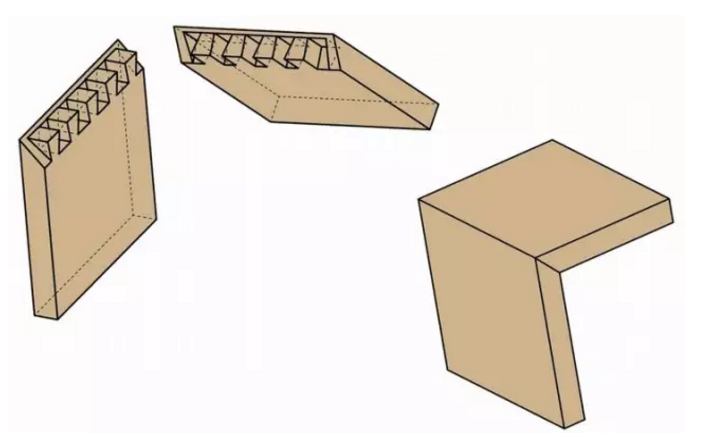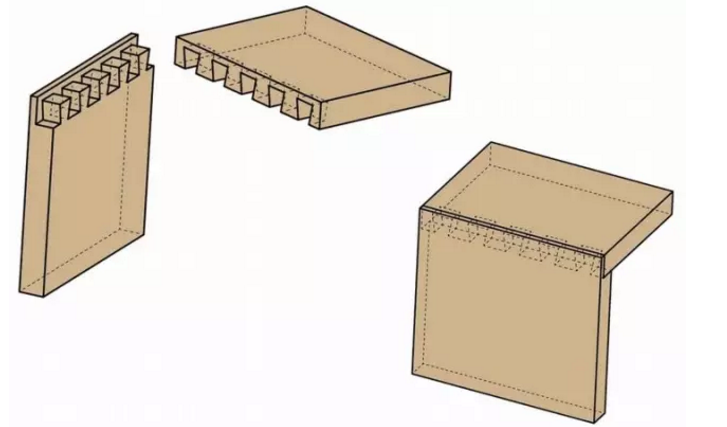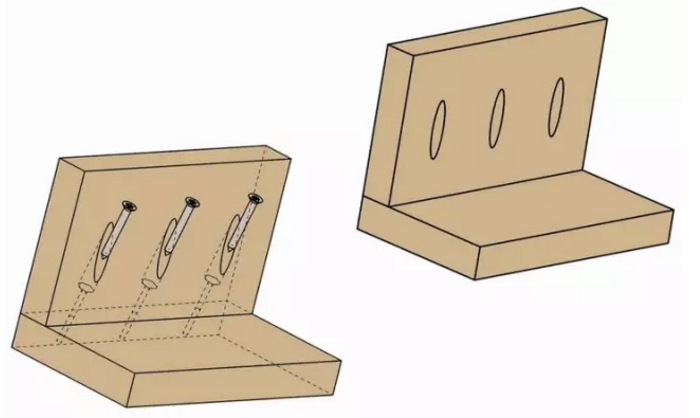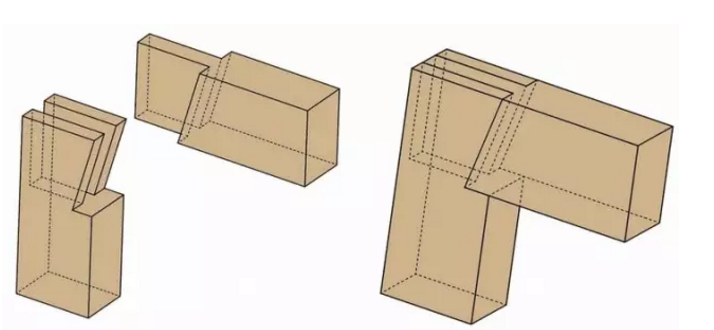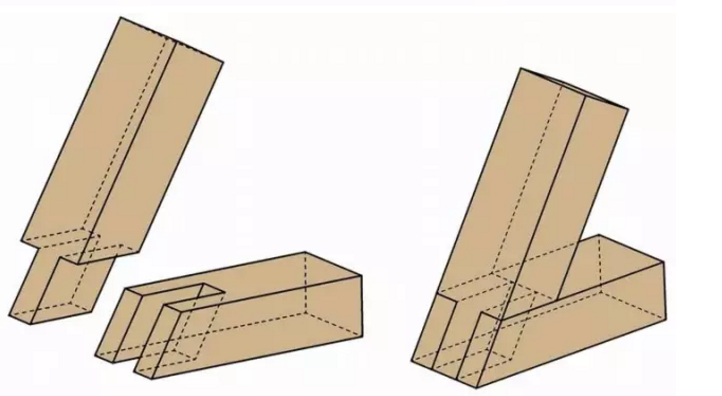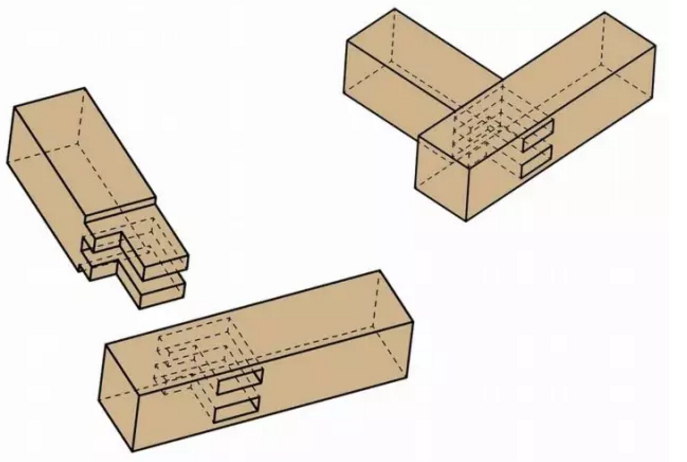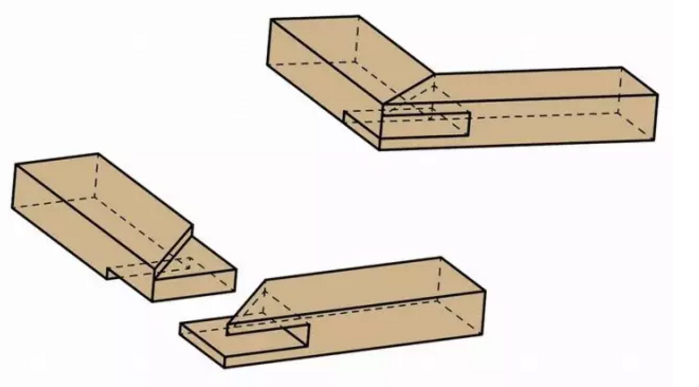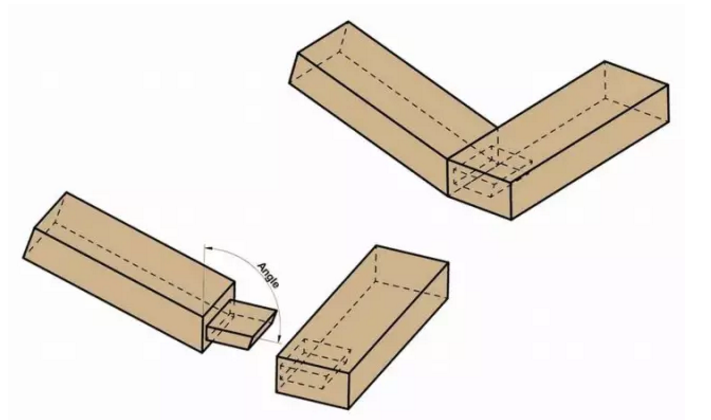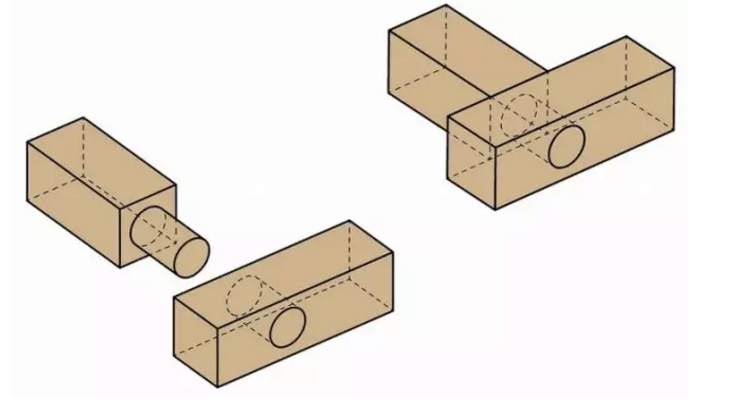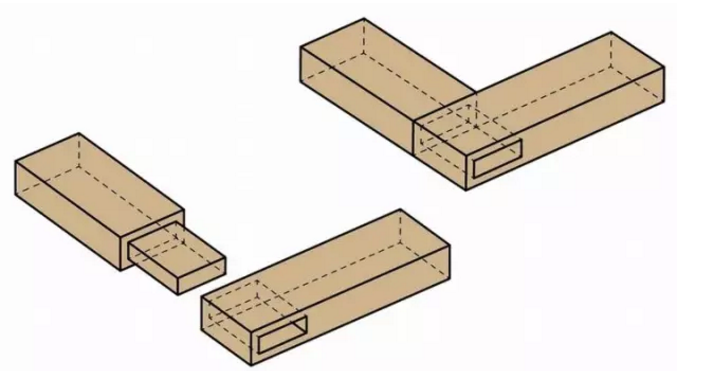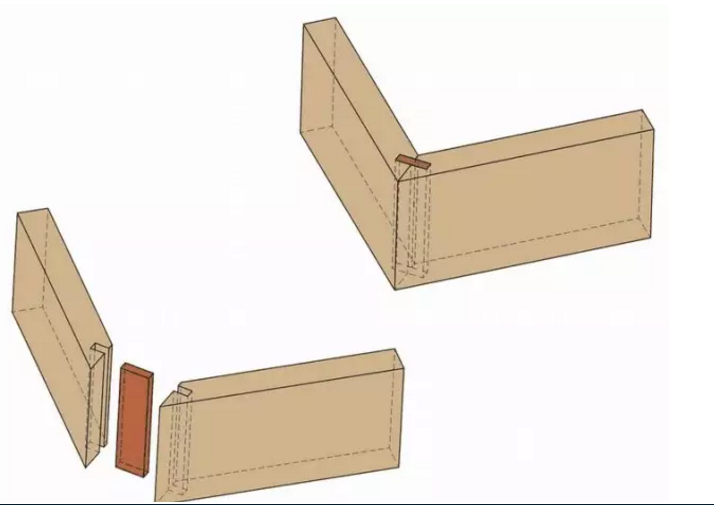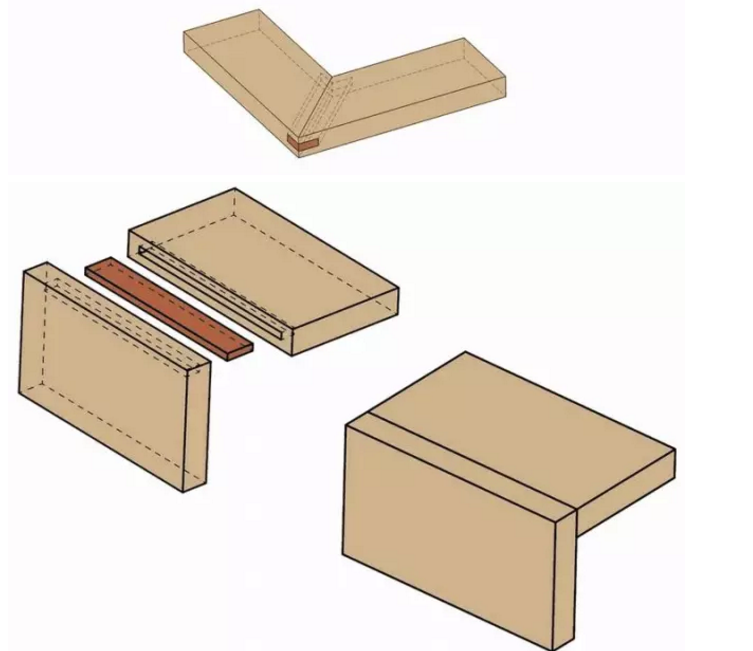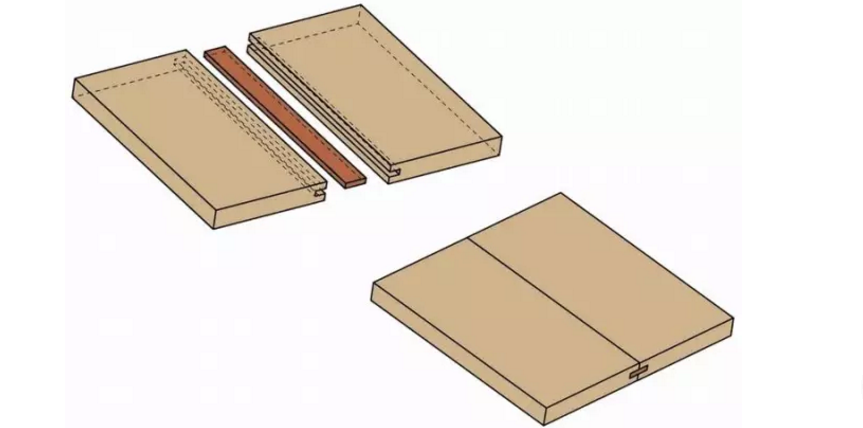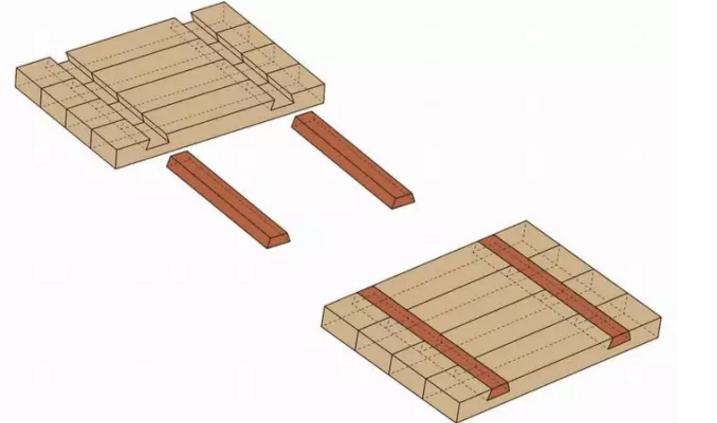饼干榫：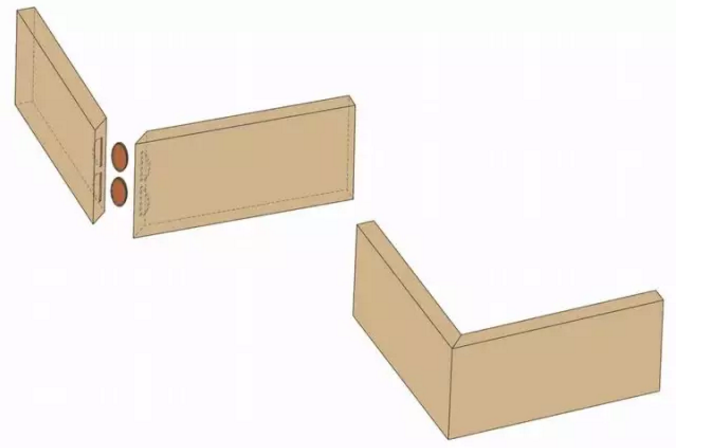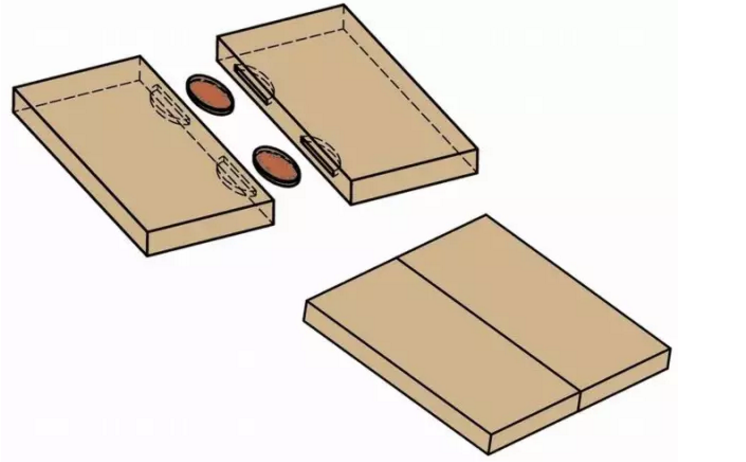### 相关资讯更多>>

0931-5322748